# Algebra 1 : How to add integers

## Example Questions

← Previous 1 3 4 5 6 7 8

### Example Question #1 : How To Add Integers

Solve the expression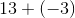.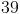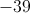Explanation:

Adding a negative is the same as subtraction.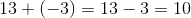### Example Question #1 : How To Add Integers

What is the sum of all of the even integers from 2 to 2,000, inclusive?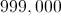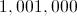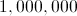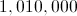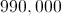Explanation:

Pair the numbers as follows: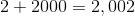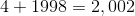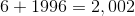...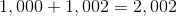There are 500 such pairs, so adding all of the even integers from 2 to 2,000 is the same as taking 2,002 as an addend 500 times. This can be rewritten as a multiplication.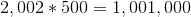### Example Question #3 : How To Add Integers

Simplify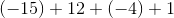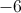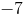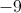Explanation:When adding integers first consider the signs of the numbers being added. Then simply work right to left.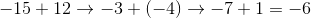So the answer is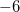### Example Question #1 : How To Add Integers

Consider the expression: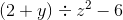If you know the value ofand, then in which order would you carry out the four operations in this expression in order to evaluate it?

Explanation:

According to the order of operations:

Work any operation in parentheses first - here it would be the addition.

What remains would be an exponent (the squaring), a division, and a subtraction, which, according to the order of operations, would be worked in that order.

### Example Question #5 : How To Add Integers

The Venn diagram depicts the number of students taking Spanish and Japanese classes. How many students in total are taking Japanese class?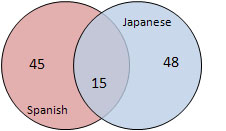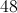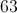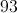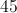Explanation:

There are 48 students taking only Japanese and 15 students taking both Japanese and Spanish. The total number taking Japanese classes is the sum of these two groups.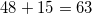### Example Question #4681 : Algebra Ii

Subtract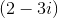from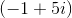.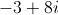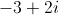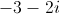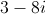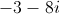Explanation:

This is essentially the following expression after translation: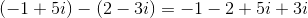Now add the real parts together for a sum of, and add the imaginary parts for a sum of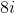.

### Example Question #61 : Imaginary Numbers

Multiply: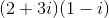Answer must be in standard form.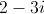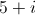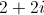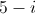Explanation:The first step is to distribute which gives us: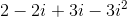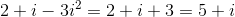which is in standard form.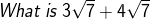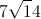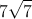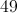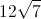Explanation: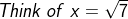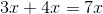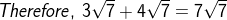### Example Question #1 : Basic Operations With Complex Numbers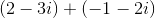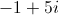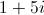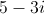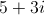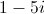Explanation:

When adding complex numbers, add the real parts and the imaginary parts separately to get another complex number in standard form.

Adding the real parts gives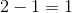, and adding the imaginary parts gives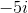.

### Example Question #1 : How To Add Integers

Solve: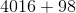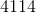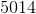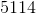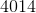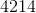Explanation:

To solve, first add the ones digit.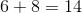The ones digit is 4. Since 14 is ten or greater, carry the tens digit to the next number.

Add the tens digit with the one carried over.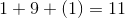The tens digit is 1.  Since 11 is ten or greater, carry the tens digit to the next number.  There are no hundreds digit with 98.  Add both the thousands and hundreds digit to the carry over.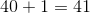Combine this with the tens and ones digits.

The answer is:← Previous 1 3 4 5 6 7 8

### All Algebra 1 Resources